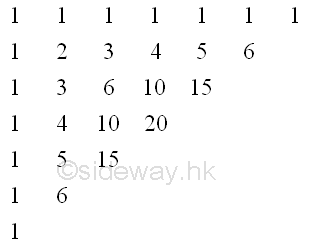output.to from Sideway
Algebra

Total Possible Arrangement

Draft for Information Only

# Content

`Total Number of Possible Arrangement Pascal's Formula Binomial Expansion Pascal's Triangle Multinomial Expansion Number of Subsets of a Set Total Possible Arrangements Finite Differences`

# Total Number of Possible Arrangement

## Pascal's Formula

Pascal's Formula, also called Pascal's Rule is a combinatiorial identity. ```Definition (Pascal's Formula) For the 𝑟-elemnet subsets of an 𝑛 element set, the following combinatorial relationship holds: 𝐶(𝑛,𝑟)=𝐶(𝑛−1,𝑟)+𝐶(𝑛−1,𝑟−1) ``` ```Proof 𝐶(𝑛,𝑟)=𝑛(𝑛−𝑟)!𝑟!  =(𝑛−1)!𝑛(𝑛−𝑟)!𝑟!  =(𝑛−1)!(𝑛−𝑟)(𝑛−𝑟)!𝑟!+(𝑛−1)!𝑟(𝑛−𝑟)!𝑟!  =(𝑛−1)!(𝑛−𝑟−1)!𝑟!+(𝑛−1)!(𝑛−𝑟)!(𝑟−1)!  =(𝑛−1)!((𝑛−1)−𝑟)!𝑟!+(𝑛−1)!((𝑛−1)−(𝑟−1)!(𝑟−1)!  =𝐶(𝑛−1,𝑟)+𝐶(𝑛−1,𝑟−1) ```

## Binomial Expansion

Binomial Expansion is an algebraic expressions of two terms. ```Binomial ExpansionThe expansion of the binomial expression (𝑎+𝑏)𝑛 is (𝑎+𝑏)𝑛=𝐶(𝑛,𝑛)𝑎𝑛+𝐶(𝑛,𝑛−1)𝑎𝑛−1𝑏+𝐶(𝑛,𝑛−2)𝑎𝑛−2𝑏2+⋯+𝐶(𝑛,1)𝑎𝑏𝑛−1+𝐶(𝑛,0)𝑎𝑏𝑛 ```

## Pascal's Triangle

Pascal's triangle is a triangular array of the binomial coefficients.## Multinomial Expansion

Binomials are just a special case of a larger class of expressions called multinomials expressions with more than one term. The expresion (𝑎+𝑏+𝑐) is a trinomial.

## Number of Subsets of a Set

For a 10 elements set {1, 2, 3, 4, 5, 6, 7, 8, 9, 10}, there are 2^10 possible sets. In mathematics of sets, This is not a proper subset of the original set, because it contains the entire set. All other subsets, including the empty set, are considered proper subsets. Therefore, there are 2^10−1 proper subsets of {1, 2, 3, 4, 5, 6, 7, 8, 9, 10}. More generally, there are 2^𝑛 subsets of an 𝑛-elemnet set, and 2^𝑛-1 proper subsets of that 𝑛n-element set.

## Total Possible Arrangements

Example of a binomial birth-order arrangements. For a family with 𝑛 children, 𝑟 of them sons, there are 𝐶(𝑛,𝑟) different birth order arrangements. `𝑛∑𝑟=0𝐶(𝑛,𝑟)=𝐶(𝑛,0)+𝐶(𝑛,1)+⋯+𝐶(𝑛,𝑟)+⋯+𝐶(𝑛,𝑛)`

The problem of total possible arrangements can be solved by relating the combinatorial representation to the binomial expransion. `(𝑎+𝑏)𝑛=𝐶(𝑛,𝑛)𝑎𝑛+𝐶(𝑛,𝑛−1)𝑎𝑛−1𝑏+𝐶(𝑛,𝑛−2)𝑎𝑛−2𝑏2+⋯+𝐶(𝑛,1)𝑎𝑏𝑛−1+𝐶(𝑛,0)𝑎𝑏𝑛`

Two equations can be equated by letting 𝑎 and 𝑏 both equal 1, `2𝑛=𝐶(𝑛,𝑛)+𝐶(𝑛,𝑛−1)+𝐶(𝑛,𝑛−2)+⋯+𝐶(𝑛,1)+𝐶(𝑛,0)`

Therefore `𝑛∑𝑟=0𝐶(𝑛,𝑟)=2𝑛` And the sum of the entries in the 𝑛th row of Pascal's triangle is 2𝑛.

```Total Possible ArrangementsThe total number of possible ways to arrange 𝑛 objects with first type of object from 0 to 𝑛 and second type of object from 𝑛 to 0 is 𝑛∑𝑟=0𝐶(𝑛,𝑟)=𝐶(𝑛,0)+𝐶(𝑛,1)+⋯+𝐶(𝑛,𝑟)+⋯+𝐶(𝑛,𝑛)=2𝑛``` Or ```Total Possible ArrangementsGiven 𝑛 objects of 𝑟 different types, then there are 𝑟𝑛 total possible ways to arrange the 𝑛 objects given all possible ways to group the 𝑟 types. ```

## Finite Differences

Pascal's TriangleReferences

1. B. Joseph, 1978, University Mathematics: A Textbook for Students of Science & Engineering, Blackie & Son Limited, HongKong
2. Wheatstone, C., 1854, On the Formation of Powers from Arithmetical Progressions, Proceedings of The Royal Society of London, Vol 7, p145-151,, London
3. Stroud, K.A., 2001, Engineering Mathematics, Industrial Press, Inc, NY
4. Coolidge, J.L., 1949, The Story of The Binomial Theorem, The American Mathematical Monthly, Vol 56, No.3, Mar, pp147-157ID: 190500011 Last Updated: 2019/5/11 Revision: Ref:Home (5)

Management

HBR (3)

Information

Recreation

Hobbies (7)

Culture

Chinese (1097)

English (336)

Reference (66)

Computer

Hardware (149)

Software

Application (187)

Digitization (24)

Numeric (19)

Programming

Web (618)CSS (SC)

ASP.NET (SC)

HTML

Knowledge Base

Common Color (SC)

Html 401 Special (SC)

OS (388)MS Windows

Windows10 (SC)

.NET Framework (SC)

DeskTop (7)

Knowledge

Mathematics

Formulas (8)

Number Theory (206)

Algebra (20)

Trigonometry (18)

Geometry (18)

Calculus (67)

Complex Analysis (21)

Engineering

Tables (8)

Mechanical

Mechanics (1)

Rigid Bodies

Statics (92)

Dynamics (37)

Fluid (5)

Control

Acoustics (19)

Biology (1)

Geography (1)